# 【Tensorflow基础】第六课：TensorBoard的使用

## TensorBoard简介，TensorBoard的使用，TensorBoard可视化

Posted by x-jeff on July 29, 2020

# 1.TensorBoard简介

TensorBoard是TensorFlow中的可视化工具包。

TensorBoard 提供机器学习实验所需的可视化功能和工具：

• 跟踪和可视化损失及准确率等指标
• 可视化模型图（操作和层）
• 查看权重、偏差或其他张量随时间变化的直方图
• 将嵌入投射到较低的维度空间
• 显示图片、文字和音频数据
• 剖析 TensorFlow 程序
• 以及更多功能

# 2.TensorBoard的使用

TensorBoard有很多栏目，例如：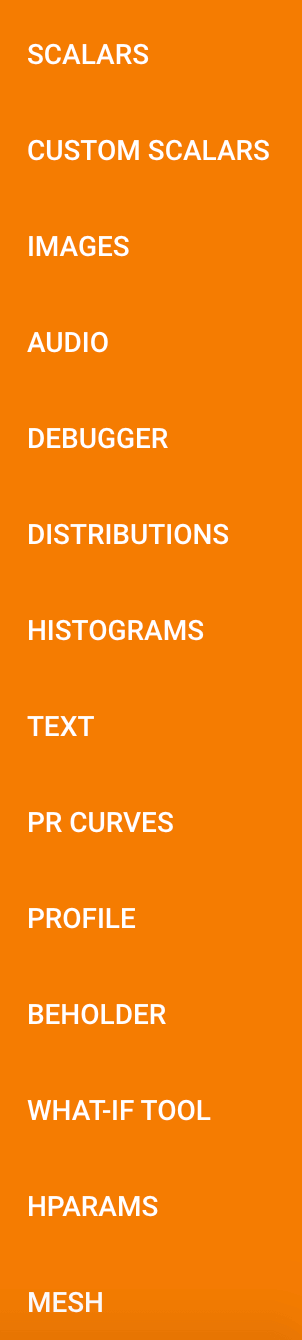## 2.1.GRAPHS

1
2
3
4
5
#命名空间
with tf.name_scope("input"):
#定义网络的输入和输出
x=tf.placeholder(tf.float32,[None,784],name='x-input')#28*28*1=784
y=tf.placeholder(tf.float32,[None,10],name='y-input')#0,1,2,3,4,5,6,7,8,9


1
writer=tf.summary.FileWriter("logs/",sess.graph)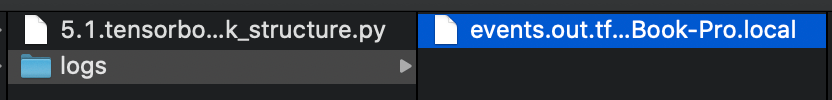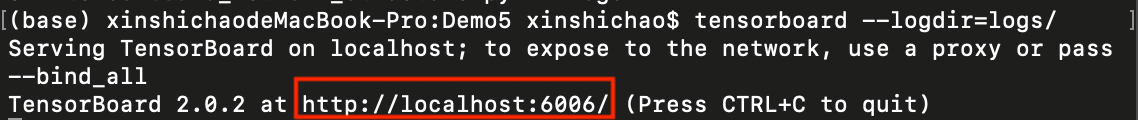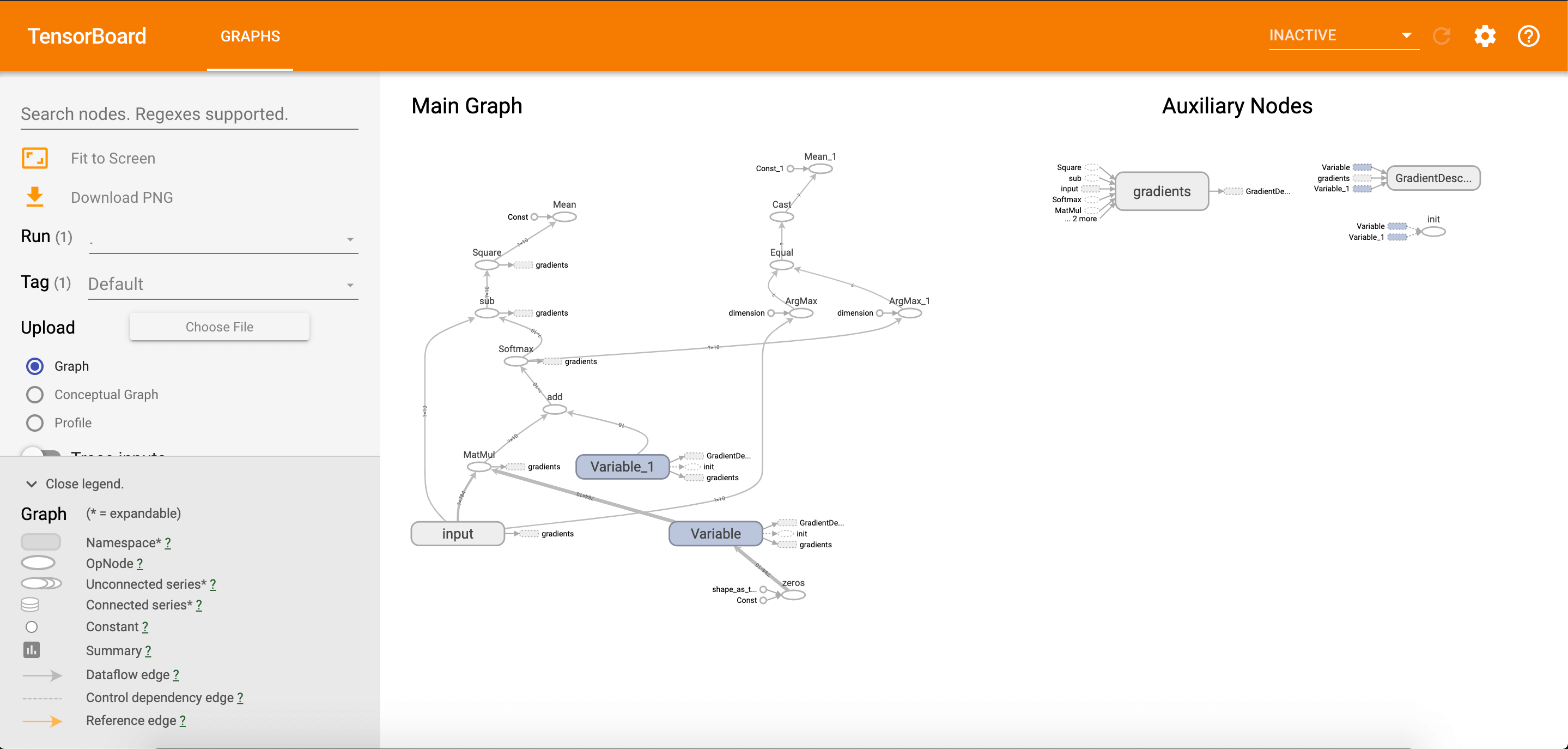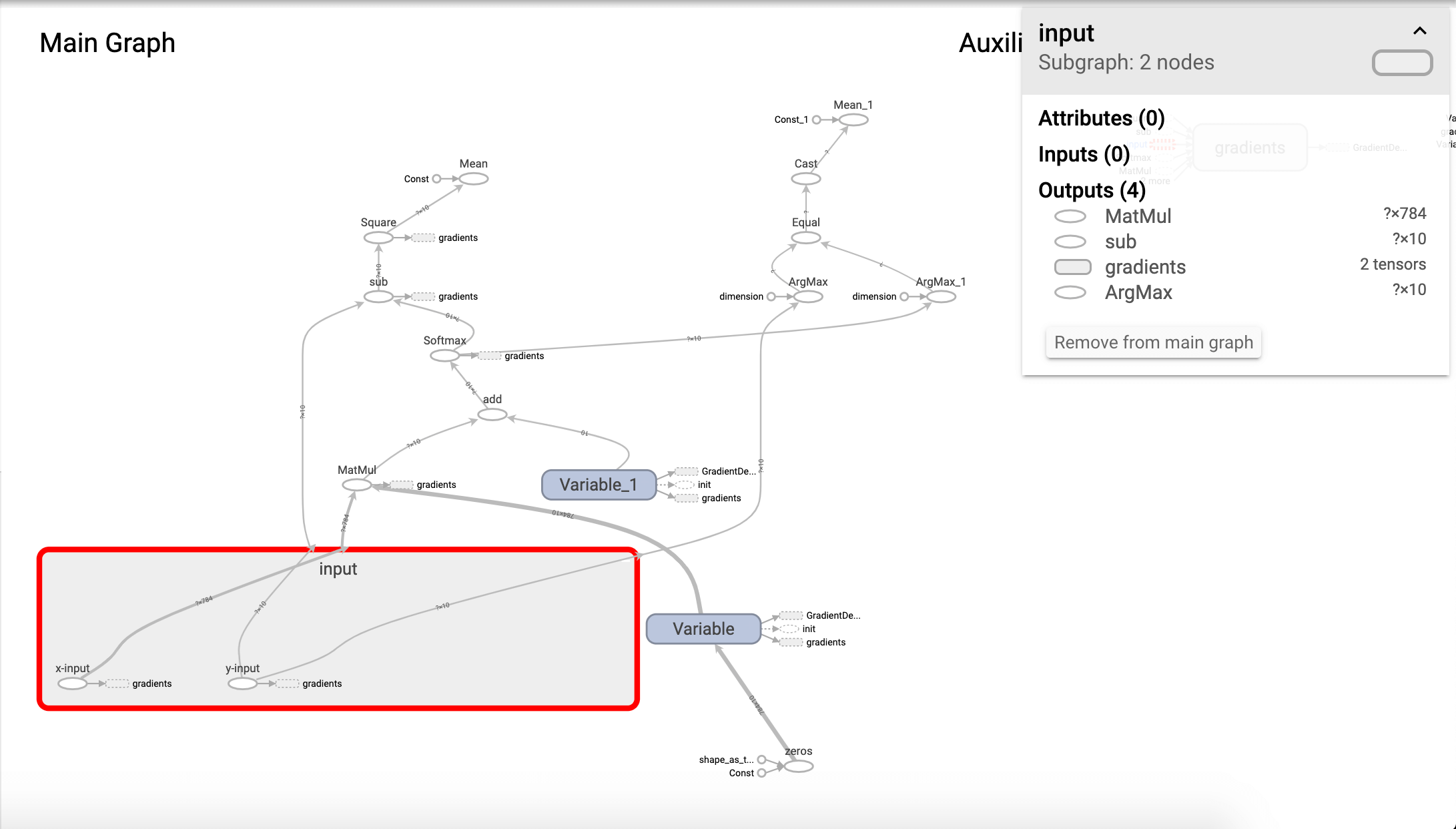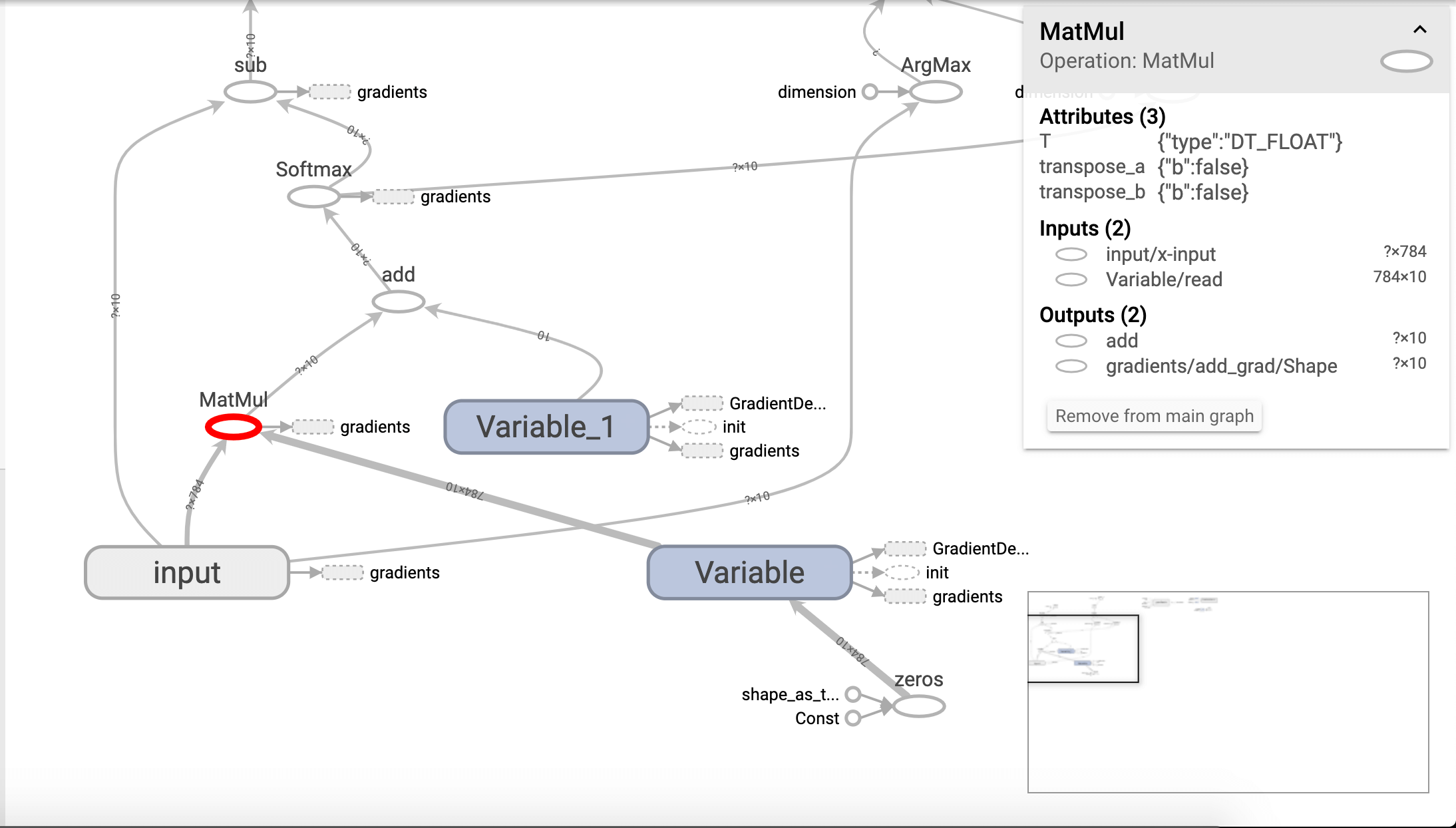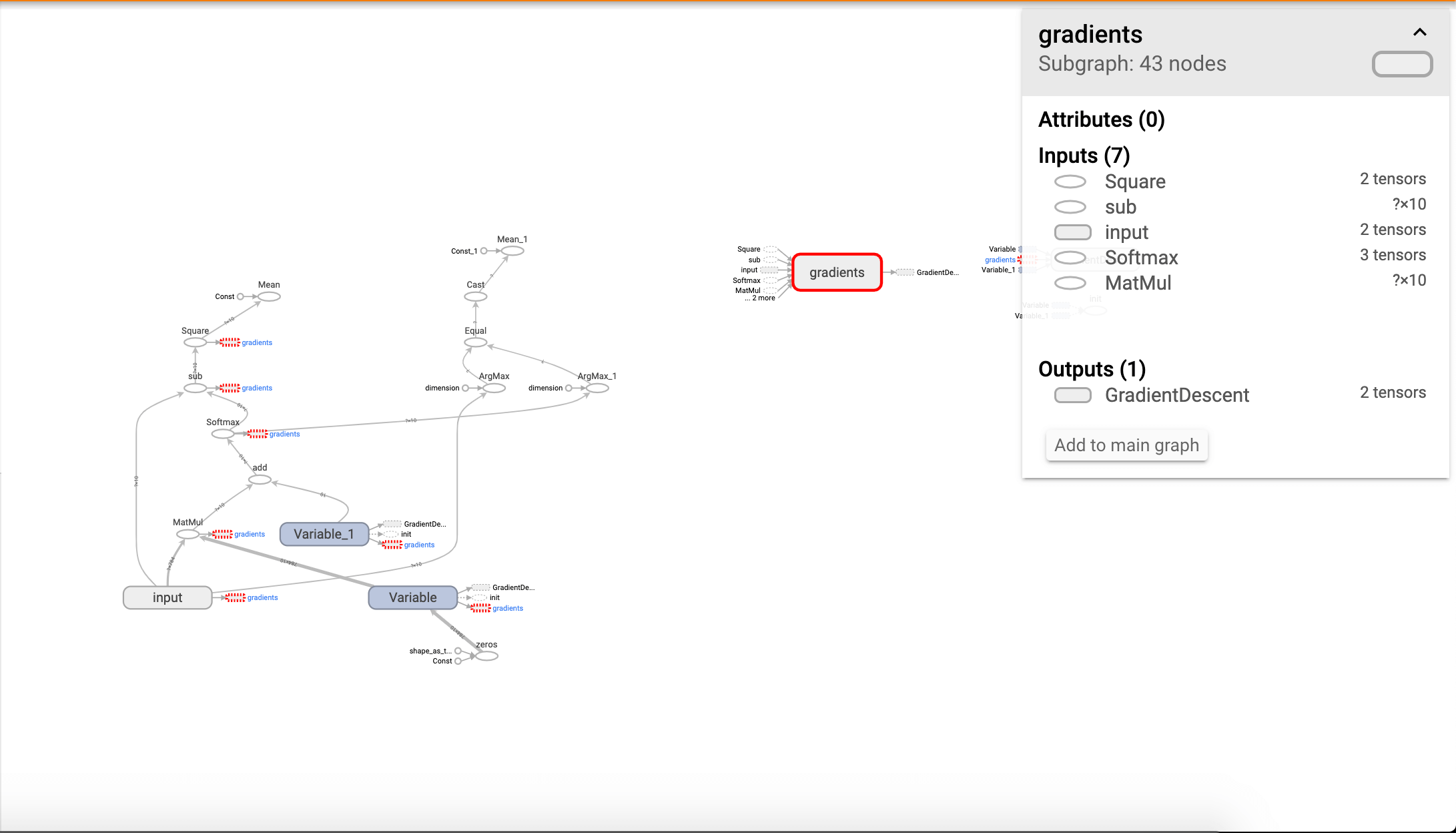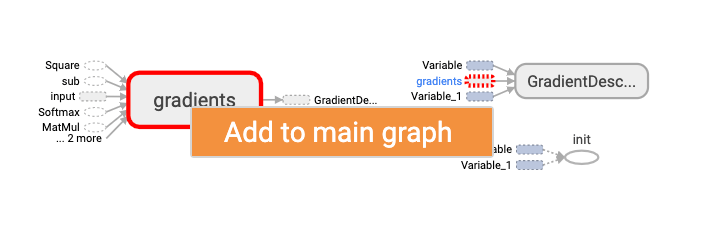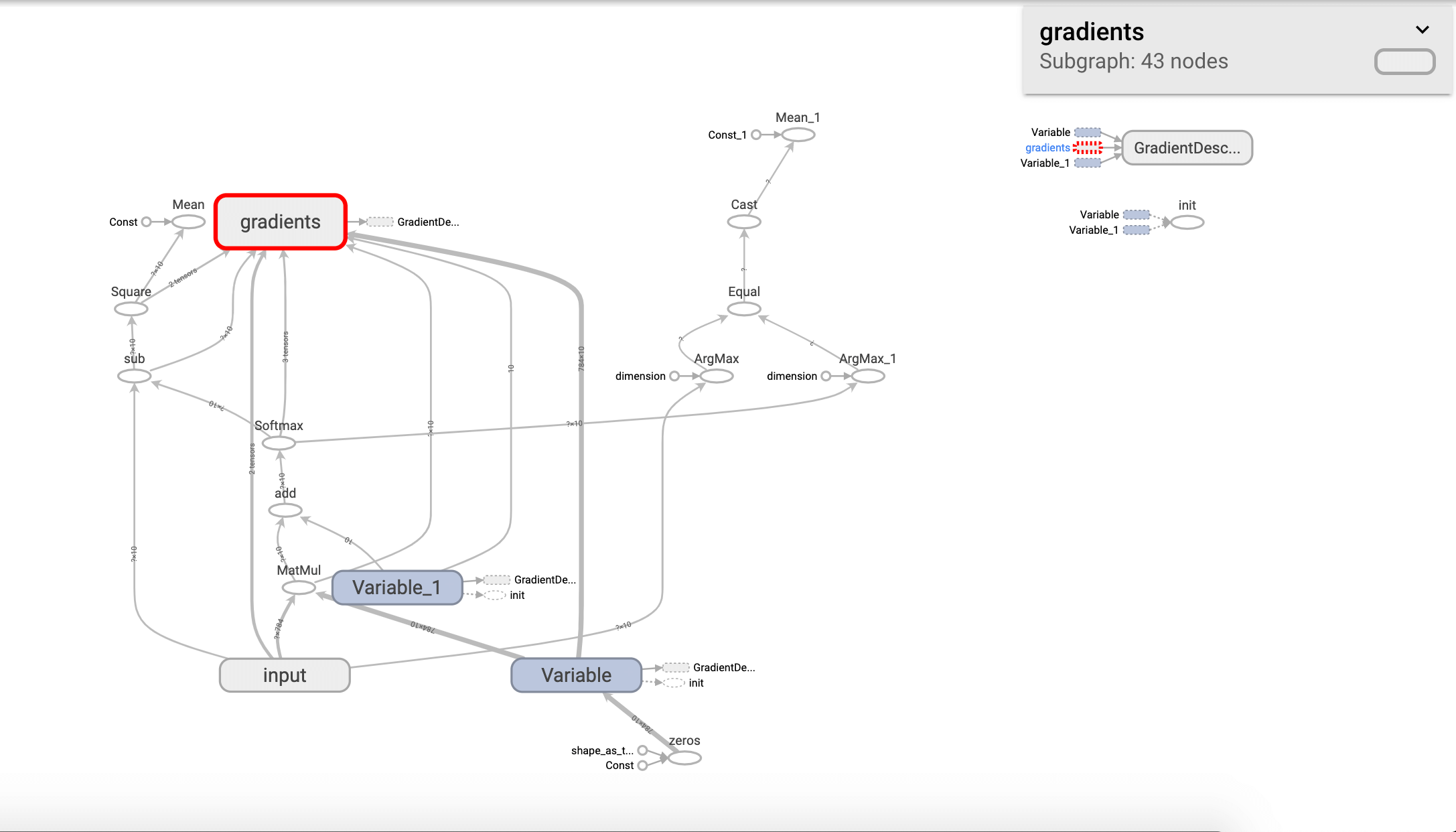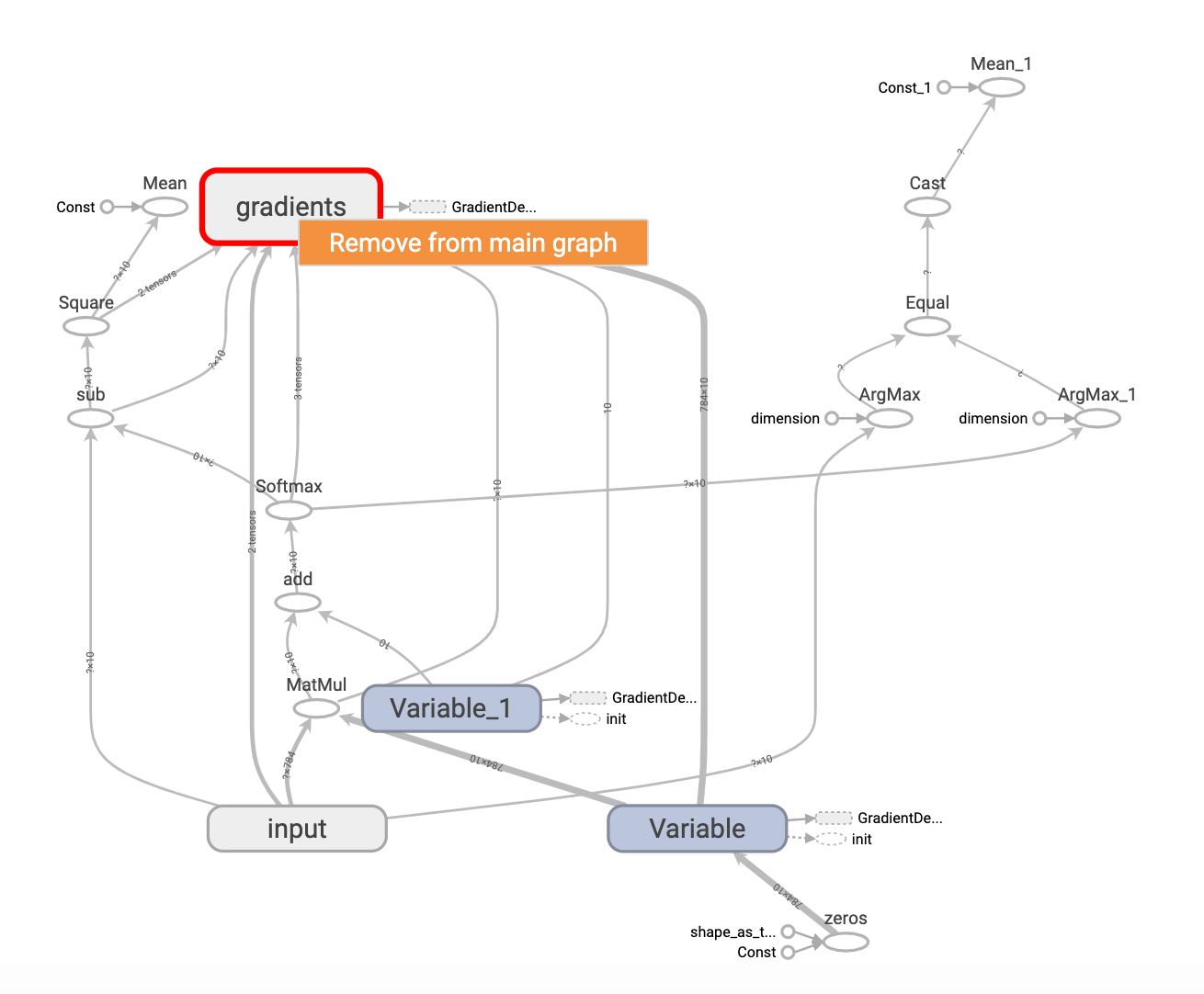1
2
3
4
5
6
7
8
9
10
with tf.name_scope("layer"):
#创建一个简单的神经网络(无隐藏层)
with tf.name_scope("weights"):
W=tf.Variable(tf.zeros([784,10]),name="W")
with tf.name_scope("biases"):
b=tf.Variable(tf.zeros(),name="b")
with tf.name_scope("wx_plus_b"):
wx_plus_b=tf.matmul(x,W)+b
with tf.name_scope("softmax"):
prediction=tf.nn.softmax(wx_plus_b)


graph中输出层结构的变化：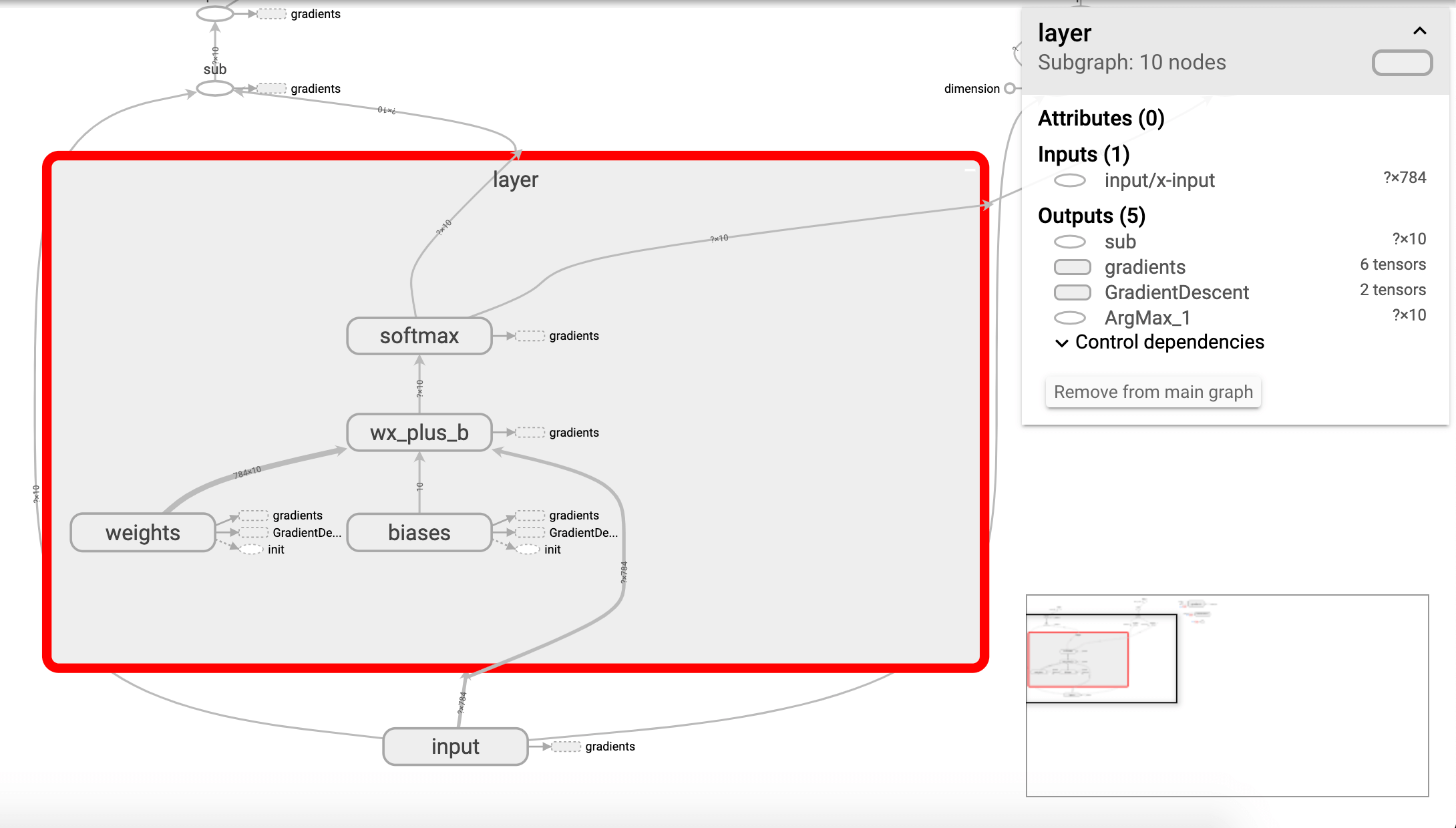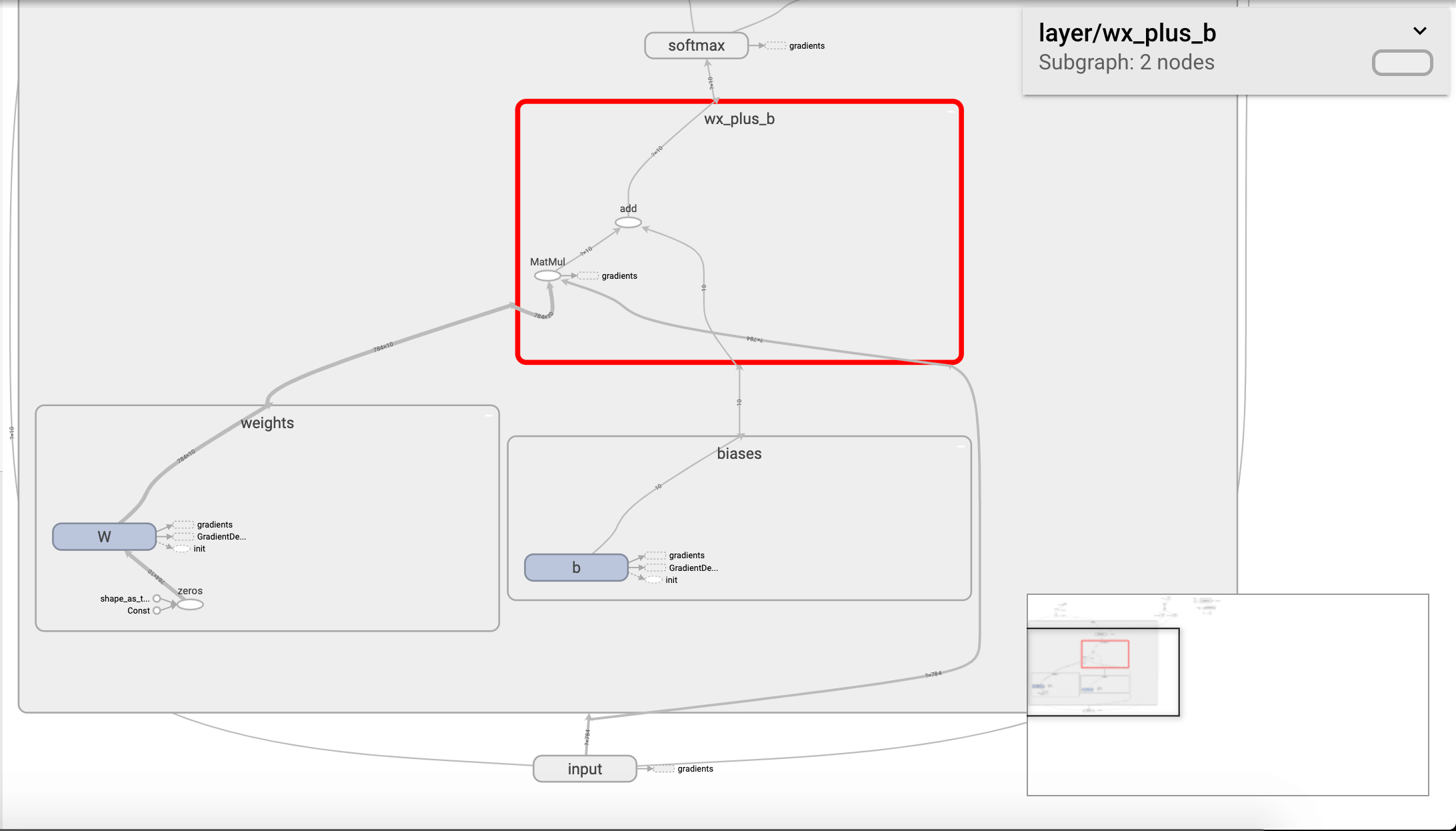1
2
3
4
5
6
7
8
9
10
11
12
13
14
15
16
with tf.name_scope("loss"):
#均方误差
loss=tf.reduce_mean(tf.square(y-prediction))
with tf.name_scope("train"):
#梯度下降法

#初始化变量
init=tf.global_variables_initializer()#有默认的init命名空间，不再额外定义命名空间

with tf.name_scope("accuracy"):
#统计预测结果
with tf.name_scope("correct_prediction"):
correct_prediction=tf.equal(tf.argmax(y,1),tf.argmax(prediction,1))#返回一个布尔型的列表
with tf.name_scope("accuracy"):
accuracy=tf.reduce_mean(tf.cast(correct_prediction,tf.float32))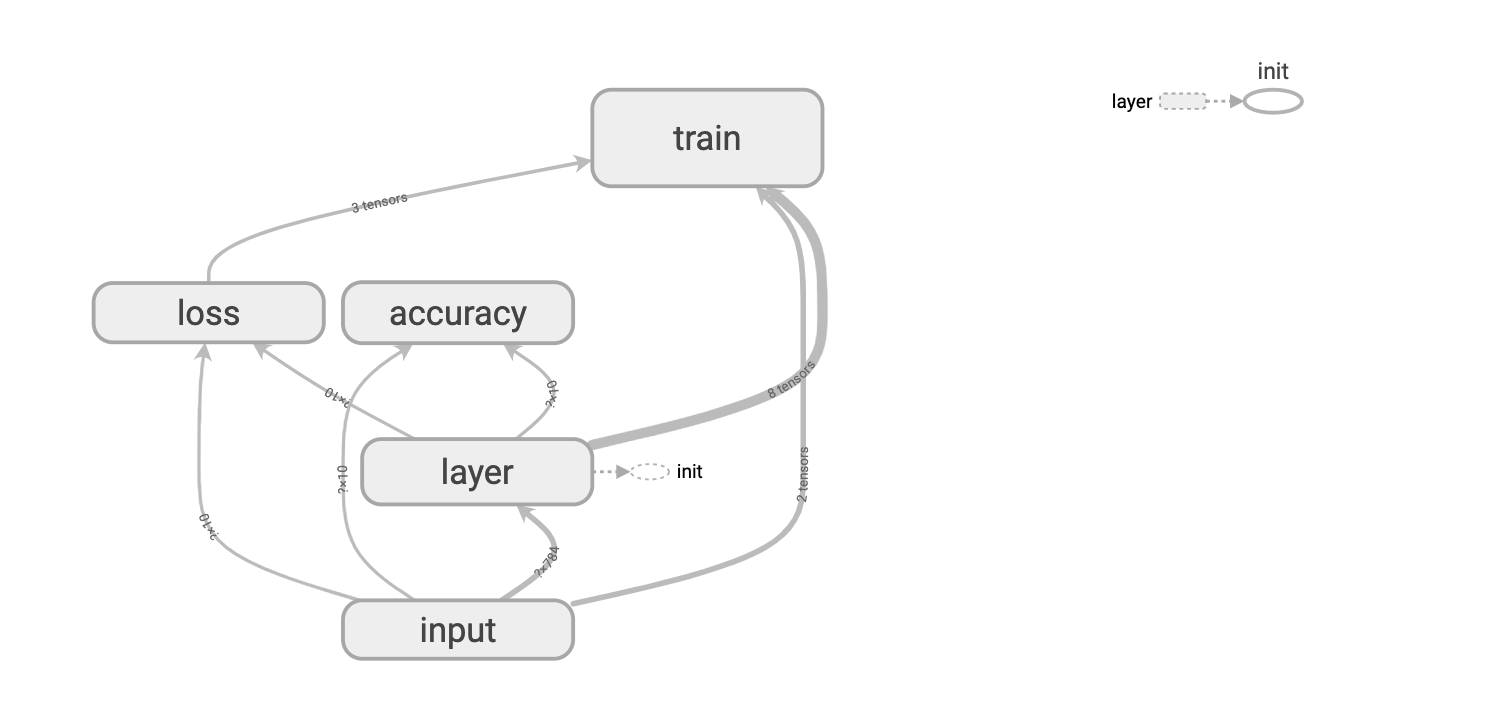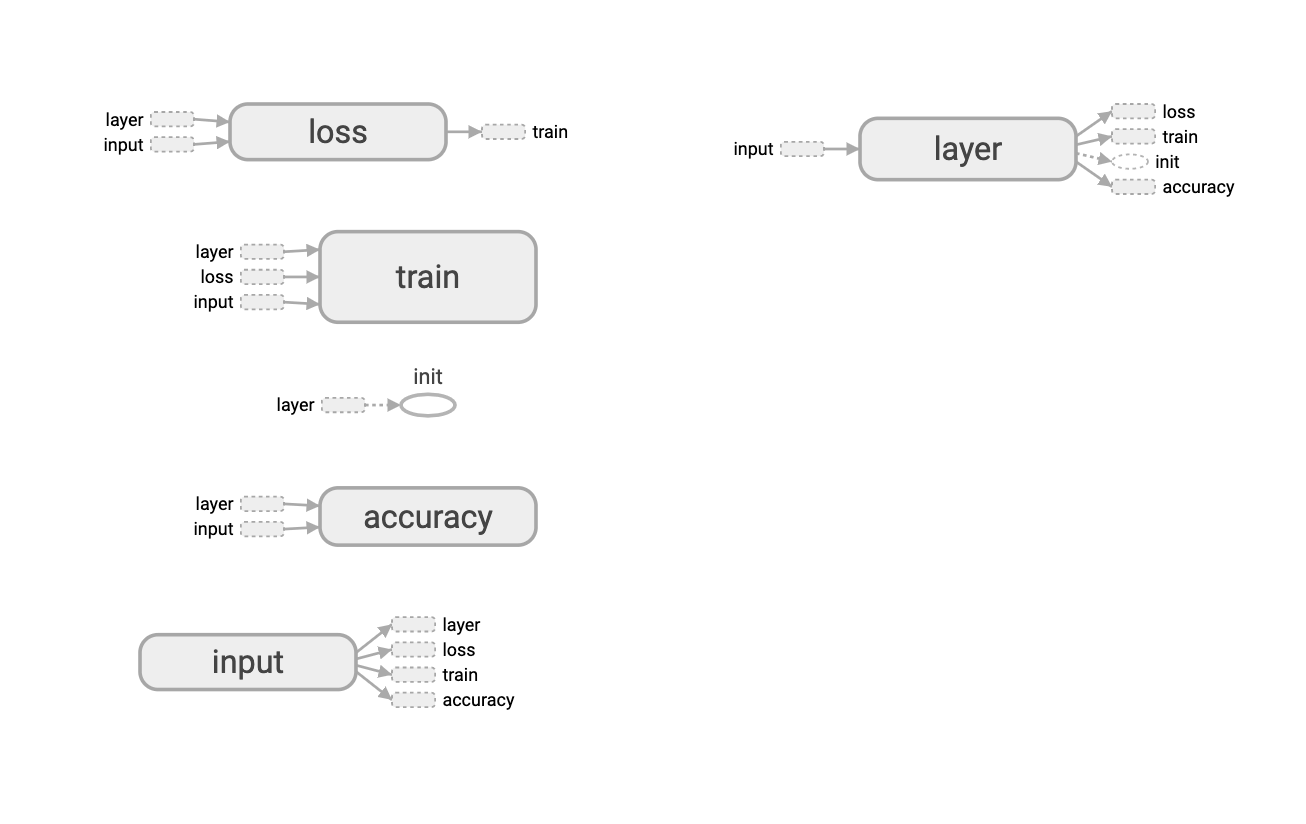## 2.2.SCALARS

tf.summary.scalar用来显示标量信息。一般在画loss,accuracy时会用到这个函数。例如，在2.1部分代码的基础上主要添加以下函数：

1
2
3
4
5
6
7
8
9
#省略
with tf.name_scope("loss"):
#均方误差
loss=tf.reduce_mean(tf.square(y-prediction))
tf.summary.scalar('loss',loss)
#省略
with tf.name_scope("accuracy"):
accuracy=tf.reduce_mean(tf.cast(correct_prediction,tf.float32))
tf.summary.scalar('accuracy',accuracy)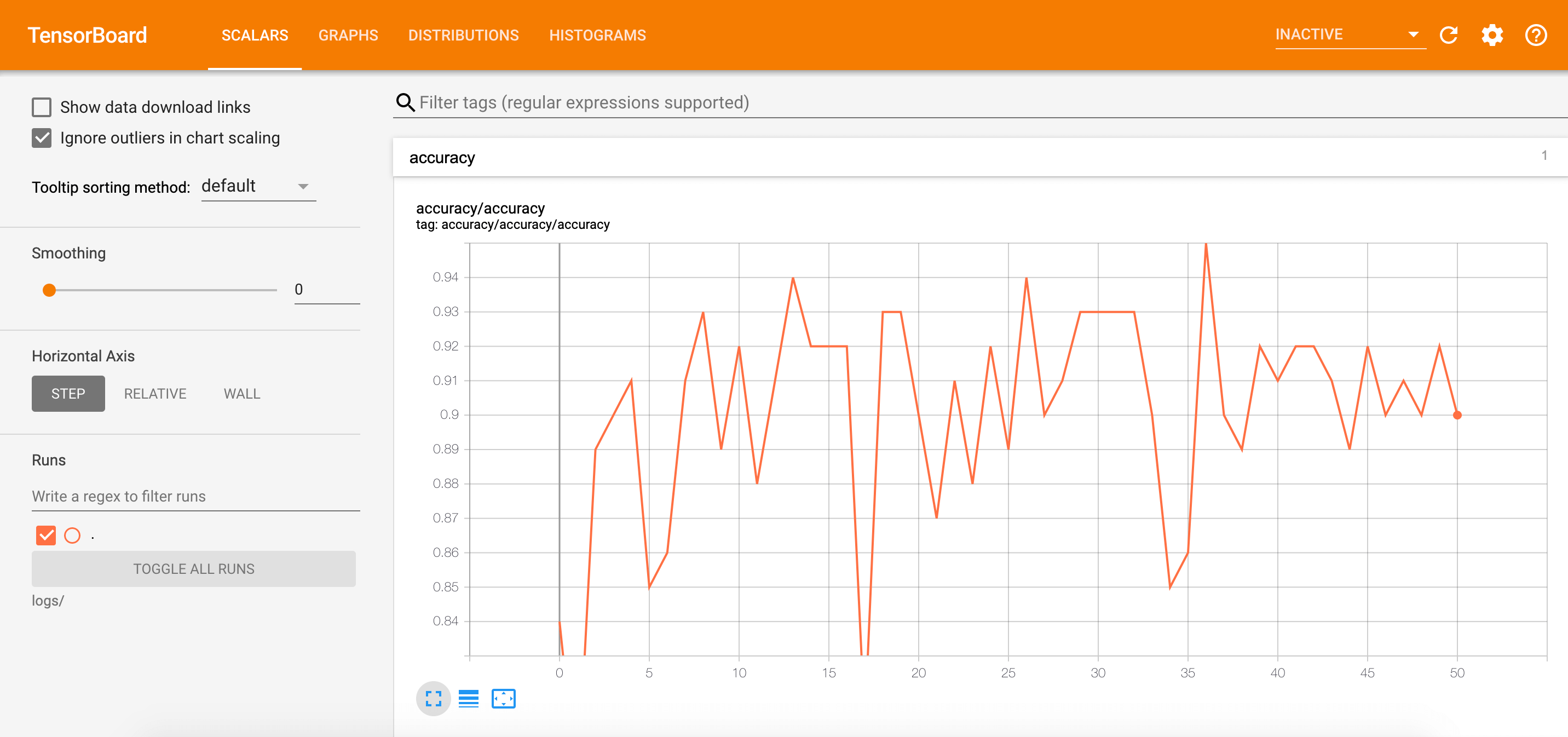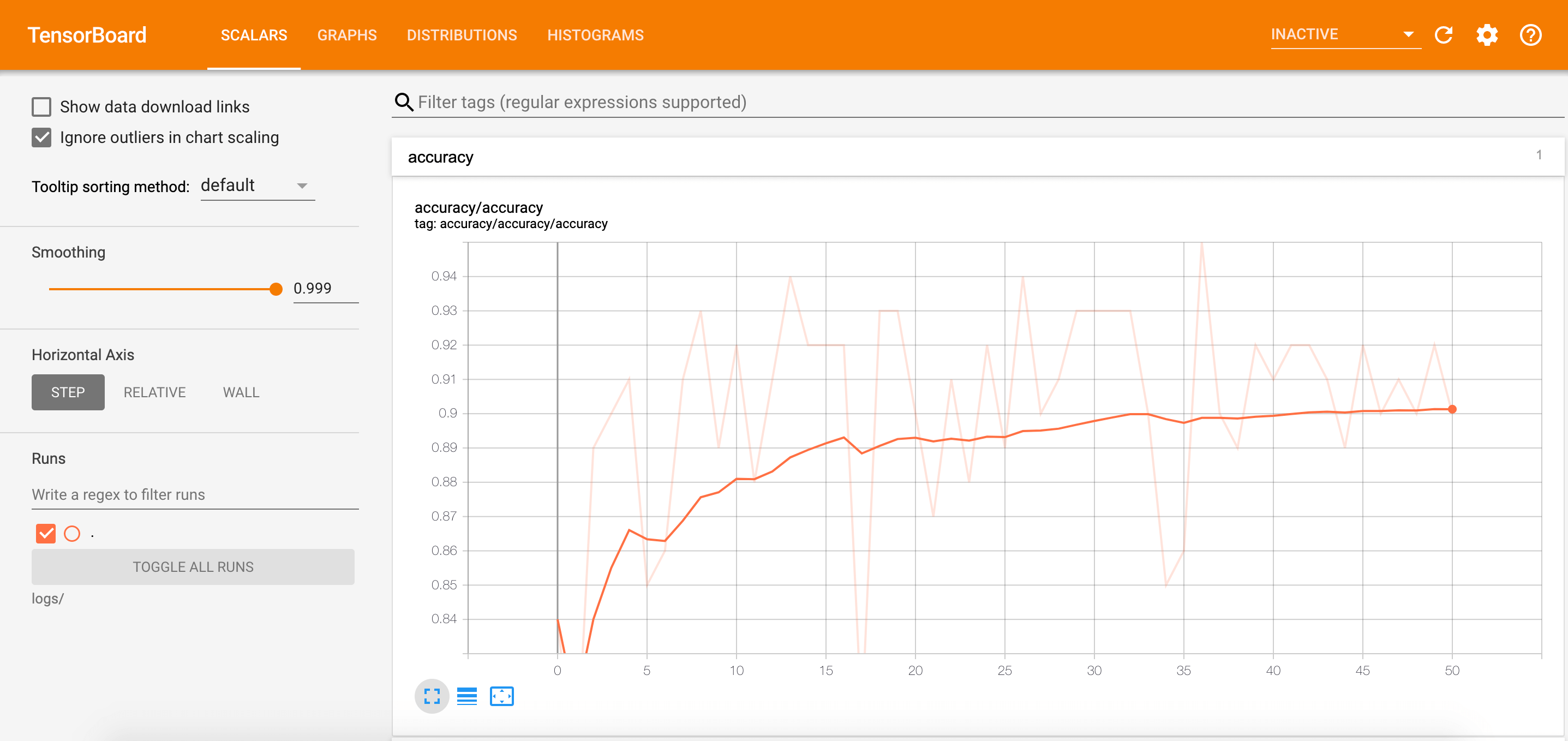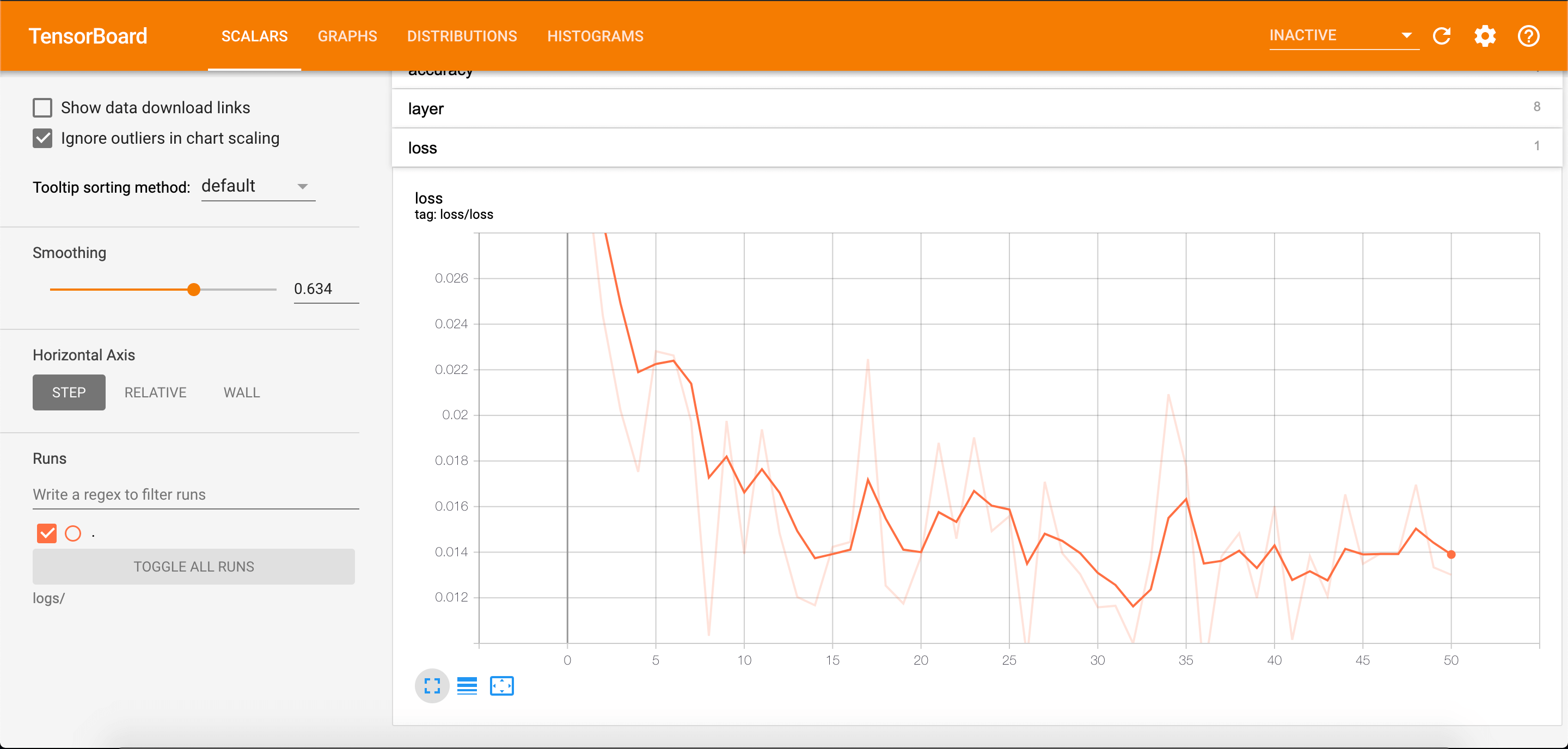## 2.3.DISTRIBUTIONS和HISTOGRAMS

HISTOGRAMSDISTRIBUTIONS这两种图的数据源是相同的，只是从不同的视角、以不同的方式来表示数据的分布情况。

1
tf.summary.histogram('histogram',var)#直方图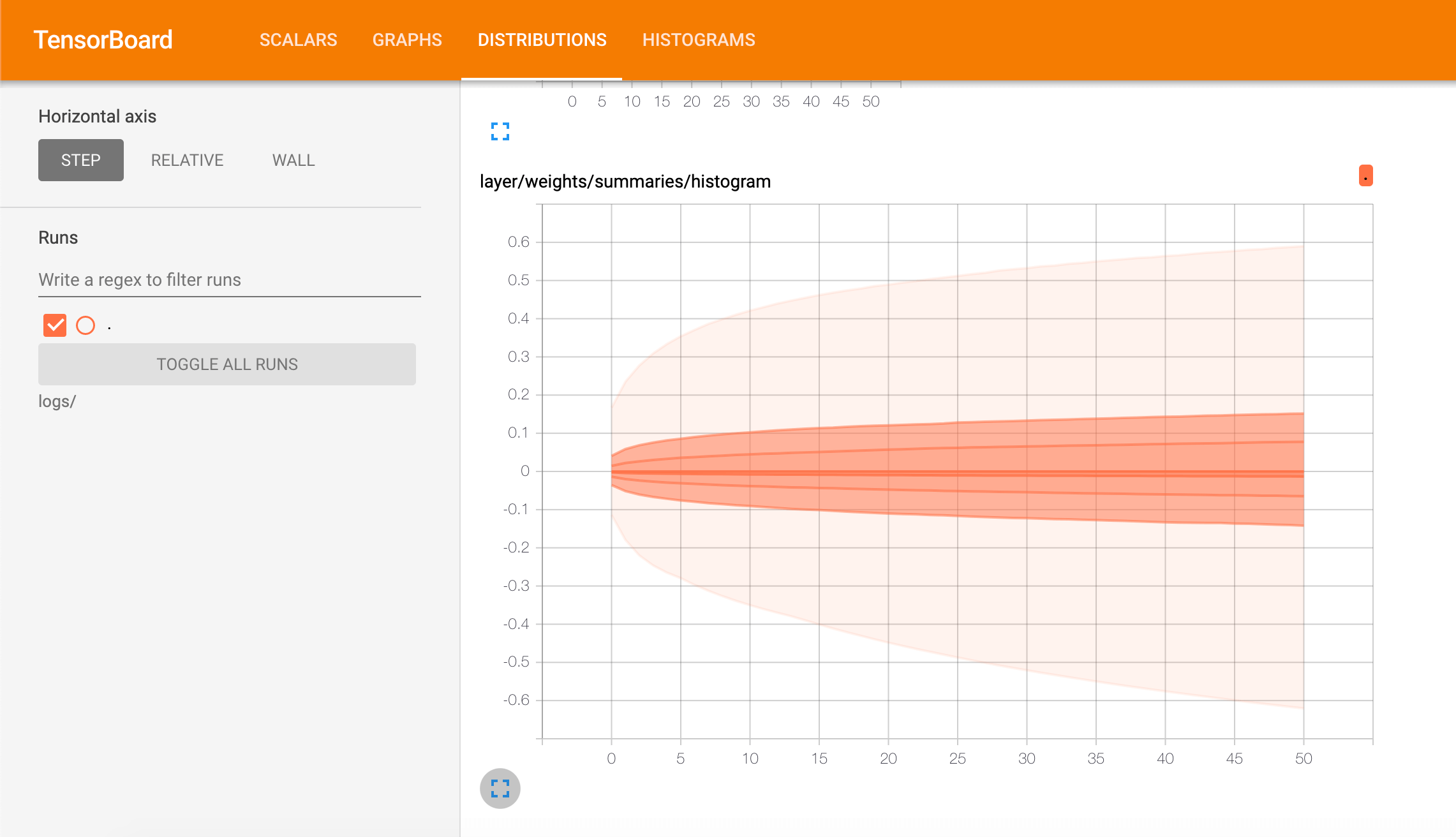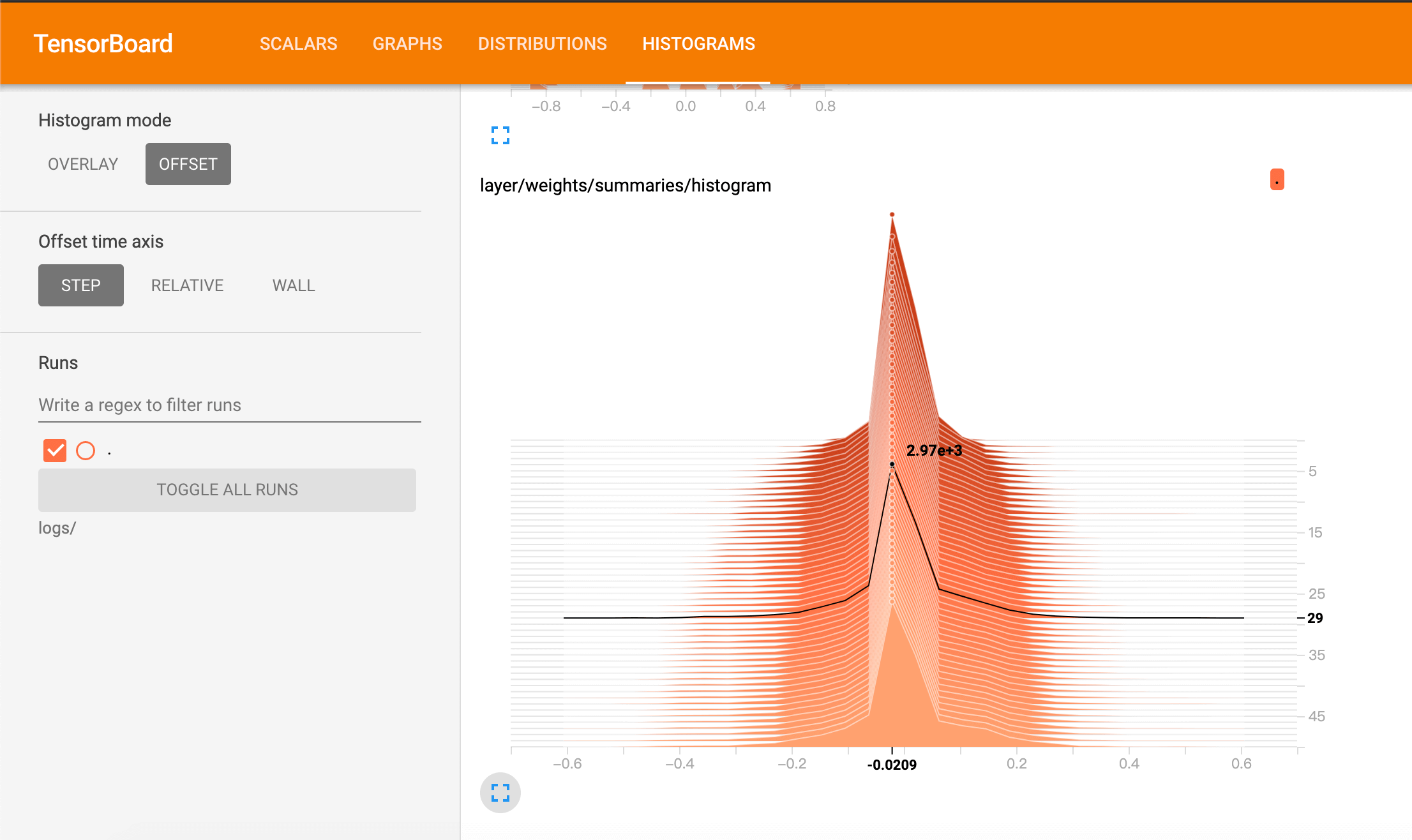# 3.TensorBoard可视化

TensorBoard提供了一个内置的交互式可视化工具：Embedding Projector。该功能用于在二维或三维空间对高维数据进行探索。

👉embedding = tf.Variable(tf.stack(mnist.test.images[:image_num]), trainable=False, name='embedding')

tf.stack()tf.unstack()

• tf.stack()是一个矩阵拼接的函数。
• tf.unstack()是一个矩阵分解的函数。
1
2
3
4
5
6
7
8
9
10
11
12
import tensorflow as tf
a=tf.constant([1,2,3])
b=tf.constant([4,5,6])
c=tf.stack([a,b],axis=0)
d=tf.stack([a,b],axis=1)
e=tf.unstack(c,axis=0)
f=tf.unstack(c,axis=1)
with tf.Session() as sess:
print("c:\n",sess.run(c))
print("d:\n",sess.run(d))
print("e:\n",sess.run(e))
print("f:\n",sess.run(f))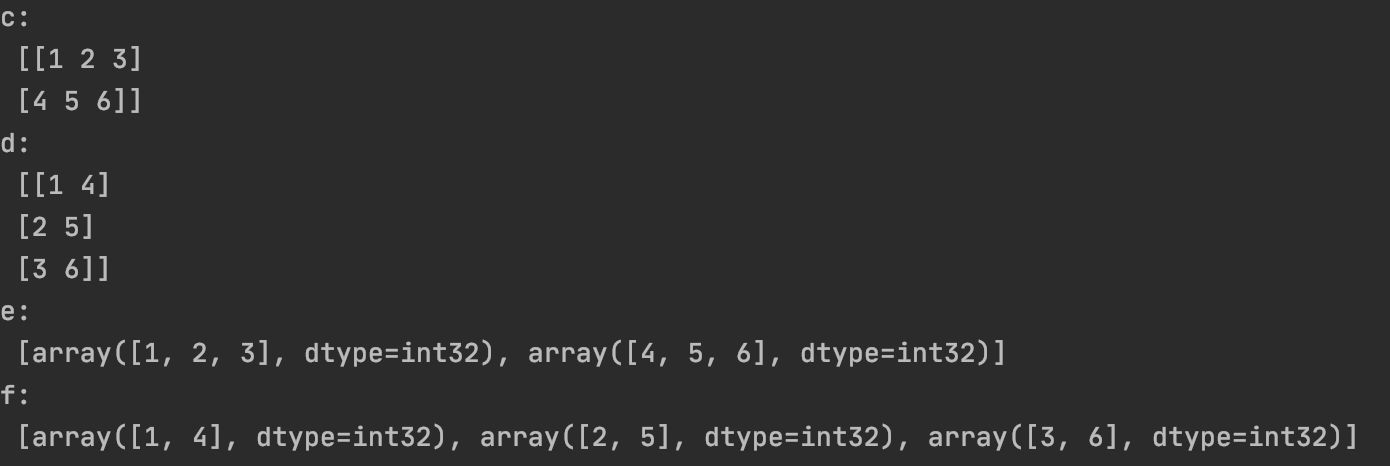mnist.test.images[:image_num]表示前image_num张测试图片。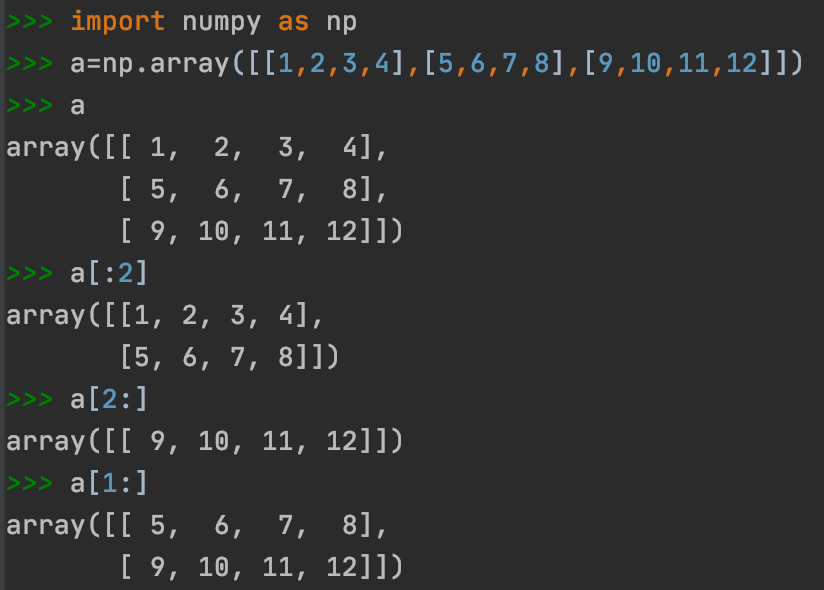trainable=False时，该变量不会被优化器更新，即无法更改。可用于定义训练过程中不用或不能被更新的参数。

👉image_shaped_input = tf.reshape(x, [-1, 28, 28, 1])

tf.reshape(tensor, shape, name=None)。其中shape为一个列表形式，特殊的一点是列表中可以存在-1。-1代表的含义是不用我们自己指定这一维的大小，函数会自动计算，但列表中只能存在一个-1。（当然如果存在多个-1，就是一个存在多解的方程了）。

👉tf.summary.image('input', image_shaped_input, 10)

tf.summary.image(name, tensor, max_outputs=3, collections=None, family=None)输出Summary带有图像的协议缓冲区。构建图像的tensor必须是4维的：[batch_size, height, width, channels]

👉tf.gfile

• tf.gfile.Exists(filename)：判断目录或文件是否存在，filename可为目录路径或带文件名的路径，有该目录则返回True，否则False。
• tf.gfile.DeleteRecursively(dirname)：递归删除所有目录及其文件，dirname即目录名，无返回。

👉保存模型：

1
2
saver = tf.train.Saver()
saver.save(sess, DIR + 'projector/projector/a_model.ckpt', global_step=max_steps)


👉可视化部分核心代码：

1
2
3
4
5
6
7
config = projector.ProjectorConfig()
embed.tensor_name = embedding.name
embed.sprite.image_path = DIR + 'projector/data/mnist_10k_sprite.png'
embed.sprite.single_image_dim.extend([28, 28])
projector.visualize_embeddings(projector_writer, config)


👉tf.RunOptions()tf.RunMetadata()：用于收集网络运行过程中的跟踪信息，包括延时，内存开销等。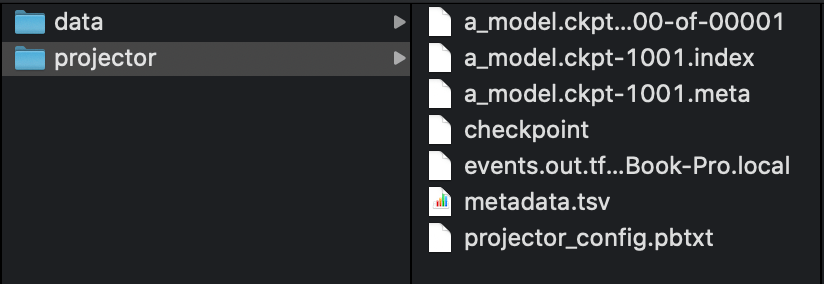1
tensorboard --logdir=projector/projector/


## 3.1.IMAGES

1
2
3
4
# 显示图片
with tf.name_scope('input_reshape'):
image_shaped_input = tf.reshape(x, [-1, 28, 28, 1])
tf.summary.image('input', image_shaped_input, 10)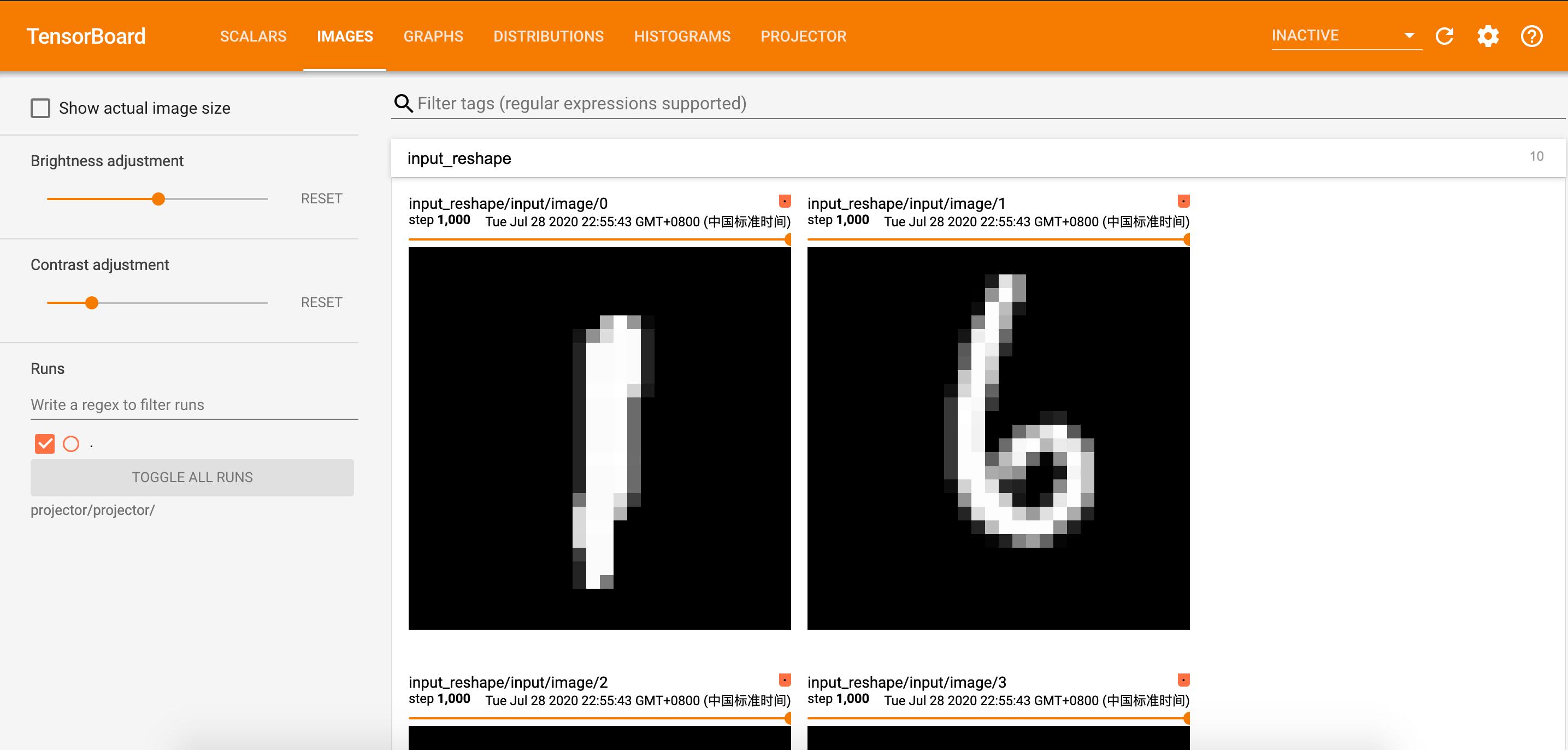## 3.2.PROJECTOR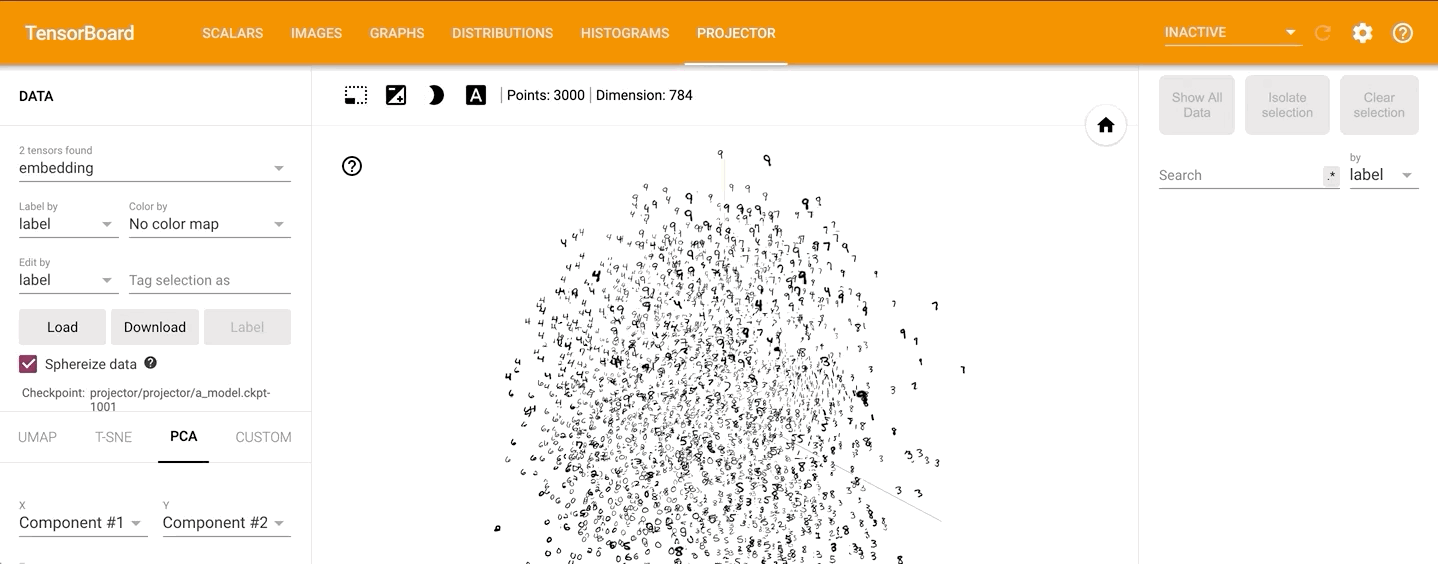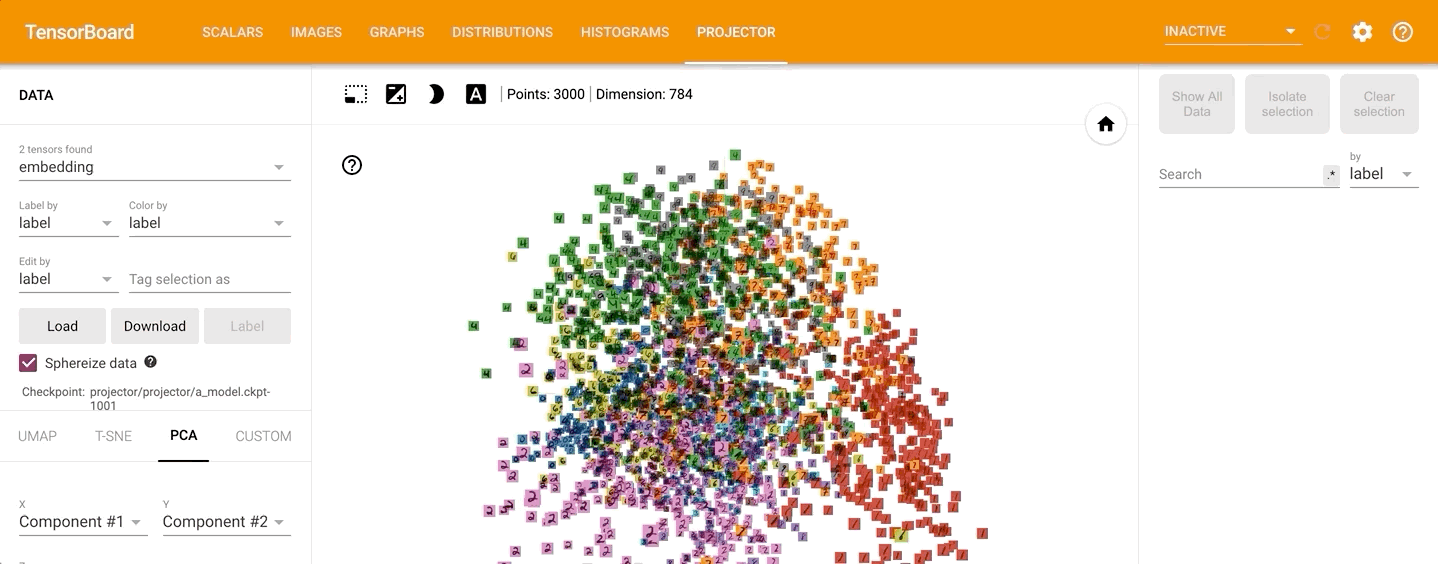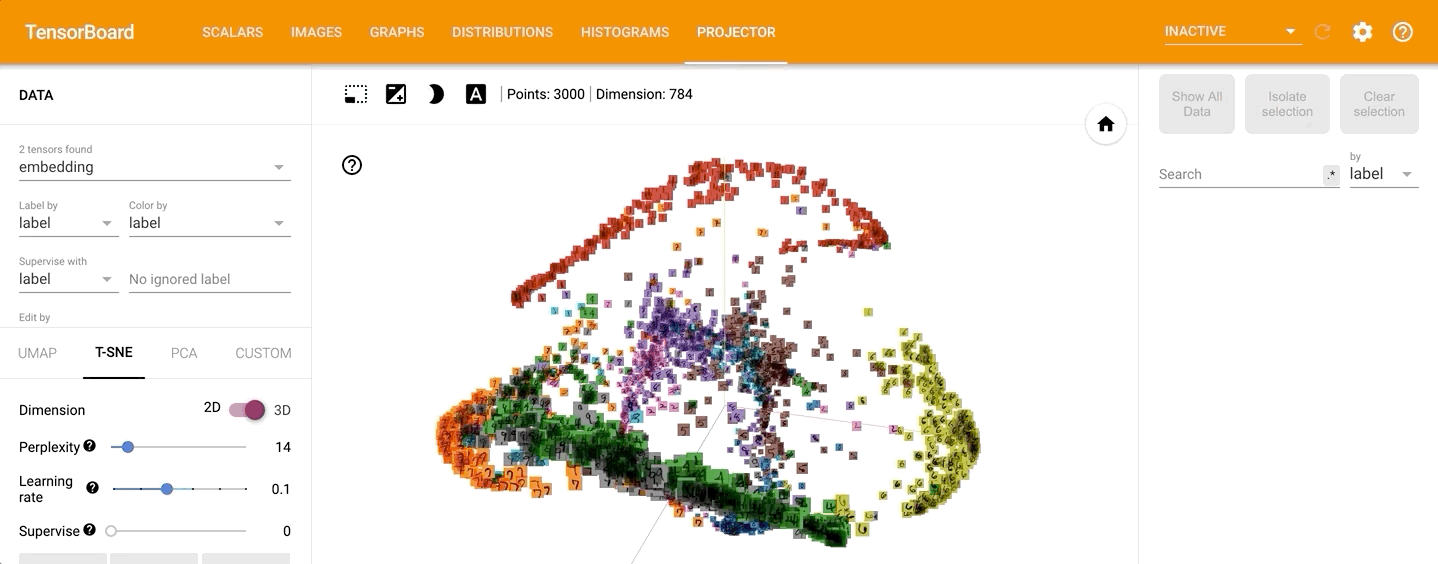# 5.参考资料